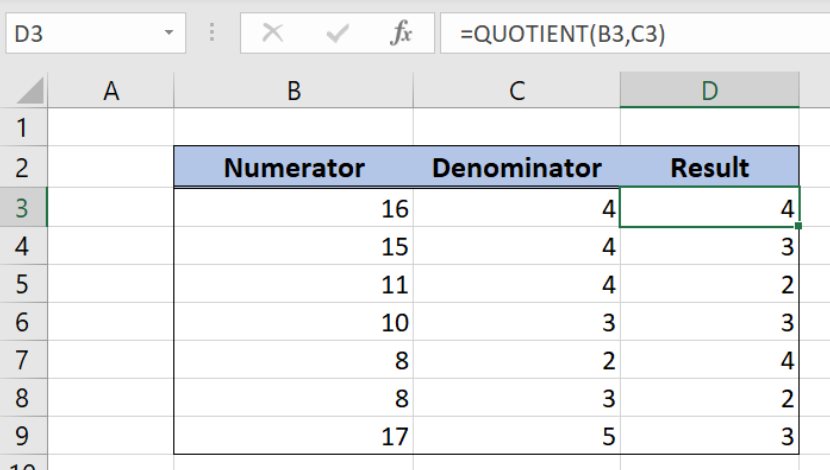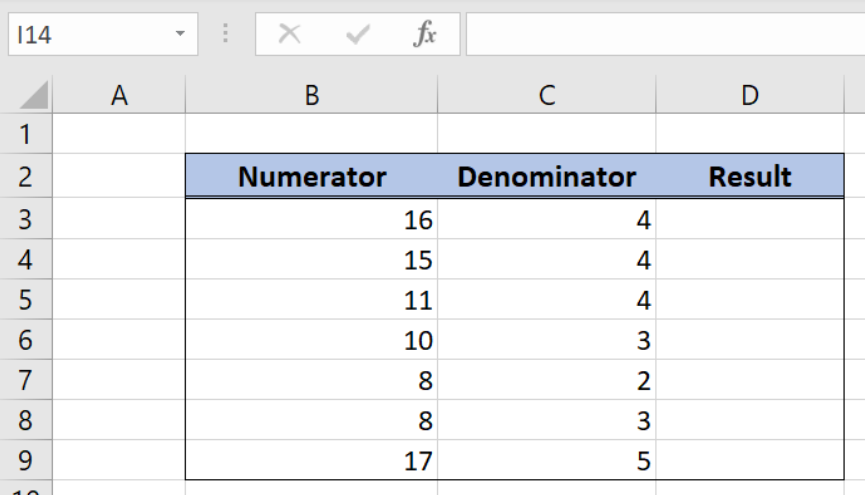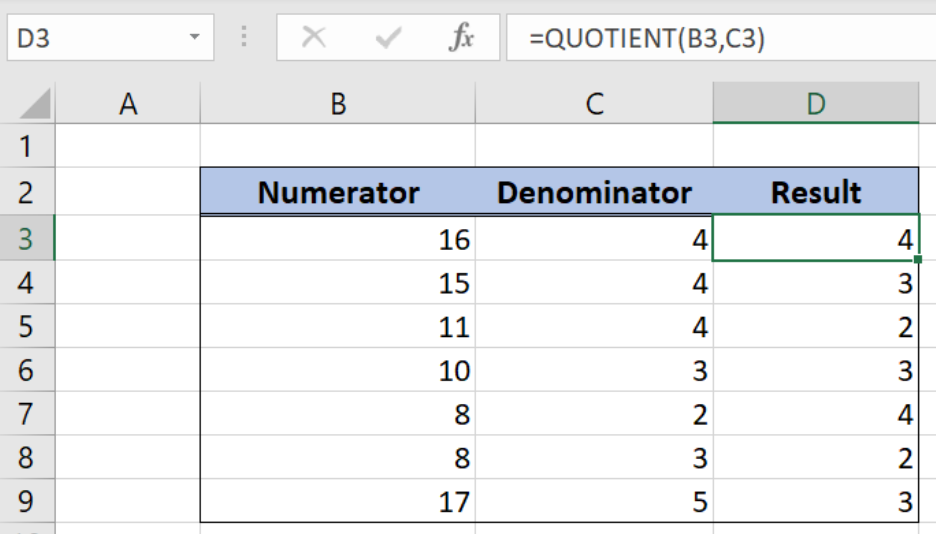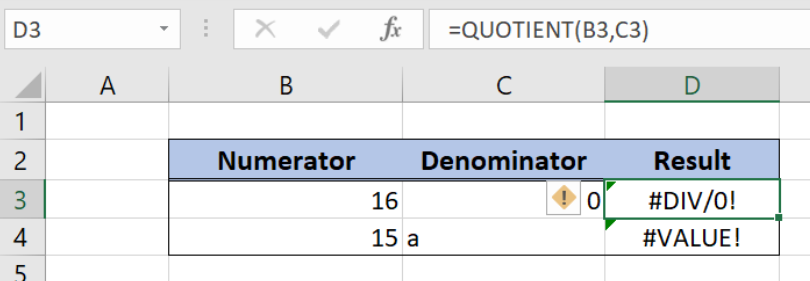Get instant live expert help with Excel or Google Sheets“My Excelchat expert helped me in less than 20 minutes, saving me what would have been 5 hours of work!”

#### Post your problem and you'll get expert help in seconds

Your message must be at least 40 characters
Our professional experts are available now. Your privacy is guaranteed.

# Excel QUOTIENT Function

Excel allows a user to get a result of division without a remainder using the QUOTIENT function. This step by step tutorial will assist all levels of Excel users in dividing without a remainder. We will also cover the common errors experienced while using the function.Figure 1. The result of the QUOTIENT function

## Syntax of the QUOTIENT Formula

The generic formula for the QUOTIENT function is:

`=QUOTIENT(numerator, denominator)`

The parameters of the PPMT function are:

• numerator – a number which we want to divide
• denominator – a divisor.

Note that both numerator and denominator need to be integers.

## Setting up Our Data for the QUOTIENT FunctionFigure 2. Data that we will use in the QUOTIENT example

Let’s look at the structure of the data we will use. The table consists of 3 columns: “Numerator” (column B), “Denominator” (column C) and “Result” (column D). We want to get the result of the QUOTIENT function in column D.

## Divide two integers without a remainder using the QUOTIENT

In our example, we want to get the principal payment for the second period in the cell E3. The interest rate is 8.50%, the total number of periods is 24 and the present value of the loan is \$8,000.

The formula looks like:

`=QUOTIENT(B3, C3)`

The parameter numerator is B3 and the parameter denominator is C3. Finally, the result in the cell D3 is 4, which is the division result without a remainder

To apply the QUOTIENT function, we need to follow these steps:

• Select cell D3 and click on it
• Insert the formula: `=QUOTIENT(B3, C3)`
• Press enter
• Drag the formula down to the other cells in the column by clicking and dragging the little “+” icon at the bottom-right of the cell.Figure 3. Using the QUOTIENT function to divide two numbers without a remainder

## Common errors when using the QUOTIENT function

There are two common errors as a result of the QUOTIENT function:

• Division by non-numeric value. If we put a character instead of an integer, we will get #VALUE! error
• Division by zero. If a denominator is 0 or empty, we will get #DIV/0! error.Figure 4. Errors when using the QUOTIENT function

Most of the time, the problem you will need to solve will be more complex than a simple application of a formula or function. If you want to save hours of research and frustration, try our live Excelchat service! Our Excel Experts are available 24/7 to answer any Excel question you may have. We guarantee a connection within 30 seconds and a customized solution within 20 minutes.